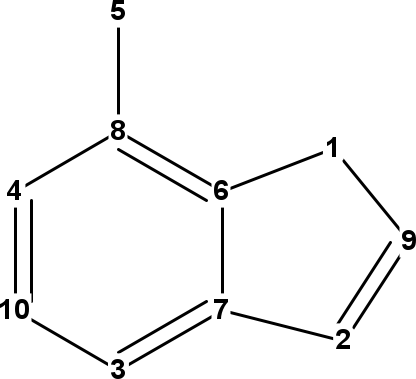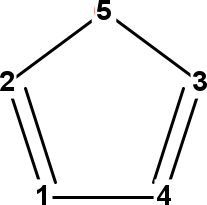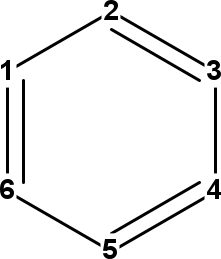# Graph Properties

Graph theory is the most common representation in cheminformatics, and with quantum mechanics, rule the informatics side of chemistry. The molecular graph follow graph theory and defines atoms as molecules and bonds as edge between to atoms. This is by far the only option, and the `IBond` allows for more complex representations, but we will focus on the molecular graph in this chapter.

## Partitioning

If one is going to calculate graph properties, the first thing one often has to do, is to split ensure that one is looking at a fully connected graph. Since this is often in combination with ensuring fully connected graphs, the `ConnectivityChecker` is a welcome tool. It allows partitioning of the atoms and bonds in an `IAtomContainer` into molecules, organized into `IAtomContainerSet`:

``````atomCon = new AtomContainer();
atom1 = new Atom("C");
atom2 = new Atom("C");
moleculeSet = ConnectivityChecker.partitionIntoMolecules(
atomCon
);
println "Number of isolated graphs: " +
moleculeSet.atomContainerCount
``````

Which gives:

``````Number of isolated graphs: 2
``````

## Spanning Tree

The spanning tree of a graph, is subgraph with no cycles; that spans all atoms into a, still, fully connected graph:

``````println "Number of azulene bonds: \$azulene.bondCount"
treeBuilder = new SpanningTree(azulene)
azuleneTree = treeBuilder.getSpanningTree();
println "Number of tree bonds: \$azuleneTree.bondCount"
``````

which returns:

``````Number of azulene bonds: 11
Number of tree bonds: 9
``````

As a side effect, it also determines which bonds are ring bonds, and which are not:

``````ethaneTree = new SpanningTree(ethane)
println "[ethane]"
println "Number of cyclic bonds: " +
ethaneTree.bondsCyclicCount
println "Number of acyclic bonds: " +
ethaneTree.bondsAcyclicCount
azuleneTree = new SpanningTree(azulene)
println "[azulene]"
println "Number of cyclic bonds: " +
azuleneTree.bondsCyclicCount
println "Number of acyclic bonds: " +
azuleneTree.bondsAcyclicCount
``````

giving

``````[ethane]
Number of cyclic bonds: 0
Number of acyclic bonds: 1
[azulene]
Number of cyclic bonds: 11
Number of acyclic bonds: 0
``````

## Ring counts

Warning: this section needs updating as there are better approaches in CDK 2.x.

Without providing a full review of all ring detection algorithms, this section discusses three algorithms for ring detection. The first two are alternative implementations of the Smallest Set of Smallest Rings (SSSR) concept. While argued to have limited usefulness if not harmful , the CDK implements two algorithms: the Figueras algorithm , and an improved algorithm addressing limitations of the first . The key limitation is that the resulting set is not unique. Depending on the initial conditions, alternative sets may result for the same structure, particularly for structures with bridgehead atoms. However, on the bright side, it does give some indication on the number of rings in a structure.

The third algorithm discussed is one to find all rings, even if they are merely combinations of smaller rings. For example, naphthalene contains has three rings.

### Smallest Rings

The smallest set of smallest rings refers to a set of unique rings that, together, capture all ring atoms. Ring atoms may participate in multiple rings in this set, but each ring will have at least one ring atom not in other rings. But for each compound, the set itself may not be unique. For example, adamantane has multiple SSSRs. The trick of the SSSR algorithm is to find a smallest set of rings that cover all ring atoms.

The CDK implements two algorithms, both are found in the `cdk.ringsearch` package. The first is the algorithm developed by Figureas  for which the `FiguerasSSSRFinder` class can be used:

``````ringset = new FiguerasSSSRFinder().findSSSR(azulene)
println "Number of rings: \$ringset.atomContainerCount"
``````

Which tells us the number of smallest rings for azulene:

``````Number of rings: 2
``````

However, because of this algorithm’s limitations in finding a correct SSSR set for some corner case structure, the following alternative method by Berger et al.  is recommended:

``````ringset = new SSSRFinder(azulene).findSSSR()
println "Number of rings: \$ringset.atomContainerCount"
``````

Which calculates the same number of rings for this compound:

``````Number of rings: 2
``````

### All Rings

If you are interesting in all possible rings, you can use the `AllRingsFinder` class, which implements an algorithm by Hanser et al. :

``````ringset = new AllRingsFinder().findAllRings(azulene)
println "Number of rings: \$ringset.atomContainerCount"
``````

Which returns all three rings present in azulene:

``````ringset = new AllRingsFinder().findAllRings(azulene)
println "Number of rings: \$ringset.atomContainerCount"
``````

One should keep in mind that when more smallest rings are fused together, the number of all rings grows quickly. The `AllRingsFinder` class has three options to keep the calculation manageable for larger structures.

The first option is to search only for rings up to a certain size. For example, only rings of at most 5 atoms:

``````ringset = new AllRingsFinder().findAllRings(azulene, 5)
println "Number of rings: \$ringset.atomContainerCount"
``````

For which there is only one in azulene:

``````Number of rings: 1
``````

A second option is more like a trick to reduce the search space, but first isolating the ring systems. There are `findAllRingsInIsolatedRingSystem` alternatives for the above two approaches.

The third option to limit the execution time is to set a time out. This ensures that a computation will return after a certain time rather than continuing indefinately:

``````ringFinder = new AllRingsFinder(AllRingsFinder.Threshold.PubChem_99)
ringset = ringFinder.findAllRings(azulene)
``````

## Graph matrices

Chemical graphs have been very successfully used as representations of molecular structures, but are not always to most suitable representation. For example, for computation of graph properties often a matrix representation is used as intermediate step. The CDK has predefined helper classes to calculate two kind of graph matrices: the adjacency matrix and the distance matrix. Both are found in the `cdk.graph.matrix` package.

The adjacency matrix describes which atoms are connected via a covalent bond. All matrix elements that link to bonded atoms are 1, and those matrix elements for disconnected atoms are 0. In mathematical terms, the adjacency matrix `A` is defined as:

Copy back in the equation. MathML?

The algorithm to calculate this matrix is implemented in the `AdjacencyMatrix` class. The matrix is calculated with the static `getMatrix(IAtomContainer)` method:

``````int[][] matrix = AdjacencyMatrix.getMatrix(ethanoicAcid)
for (row=0;row<ethanoicAcid.getAtomCount();row++) {
for (col=0;col<ethanoicAcid.getAtomCount();col++) {
print matrix[row][col] + " "
}
println ""
}
``````

This code outputs the matrix, resulting for ethanoic acid, with the atoms in the order C, C, O, and O, in:

``````0 1 0 0
1 0 1 1
0 1 0 0
0 1 0 0
``````

### Distance matrix

The distance matrix describes the number of bonds on has to traverse to get from one atom to another. Therefore, it has zeros on the diagonal and non-zero values at all other locations. Matrix elements for neighboring atoms are 1 and others are larger. The CDK uses Floyd’s algorithm to calculate this matrix , which is exposed via the `TopologicalMatrix` class:

``````int[][] matrix = TopologicalMatrix.getMatrix(ethanoicAcid)
``````

For the ethanoic acid used earlier, the resulting matrix looks like:

``````0 1 2 2
1 0 1 1
2 1 0 2
2 1 2 0
``````

## Atom Numbers

Another important aspect of the chemical graph, is that the graph uniquely places atoms in the molecule. That is, the graphs allows us to uniquely identify, and therefore, number atoms in the molecule. This is an important aspect of cheminformatics, and the concept behind canonicalization, such as used to create canonical SMILES. The InChI library (see Chapter 19) implements such an algorithm, and we can use it to assign unique integers to all atoms in a chemical graph.

### Morgan Atom Numbers

Morgan published an algorithm in 1965 to assign numbers to vertices in the chemical graph . The algorithm does not take into account the element symbols associated with those vertices, and it only based on the connectivity. Therefore, we see the same number of symmetry related atoms, even if they have different symbols. If we run:

``````oxazole = MoleculeFactory.makeOxazole();
long[] morganNumbers =
MorganNumbersTools.getMorganNumbers(
oxazole
);
for (i in 0..(oxazole.atomCount-1)) {
println oxazole.getAtom(i).symbol +
" " + morganNumbers[i]
}
``````

we see this output:

``````C 64
O 64
C 64
N 64
C 64
``````

### InChI Atom NumbersFigure 14.1: InChI atom numbers of adenine (left), oxazole (middle), and benzene (right).

The InChI library does not have a direct method to calculate atom numbers from Java, but the CDK can extract these from the auxiliary layer. These numbers are those listed in the bond layer, but to use these in the CDK molecule class, we need to mapping of the InChI atom numbers This method is made available via the `InChINumbersTools` class:

``````oxazole = MoleculeFactory.makeOxazole();
long[] morganNumbers =
InChINumbersTools.getNumbers(
oxazole
);
for (i in 0..(oxazole.atomCount-1)) {
atom = oxazole.getAtom(i)
println atom.symbol +
" " + morganNumbers[i]
}
``````

which outputs:

``````C 2
O 5
C 3
N 4
C 1
``````

It is important to note that because these numbers are used in the connectivity layer, symmetry is broken in assignment of these numbers, allowing all atoms in, for example, benzene to still be uniquely identified:

``````benzene = MoleculeFactory.makeBenzene();
long[] numbers =
InChINumbersTools.getNumbers(
benzene
);
for (i in 0..(benzene.atomCount-1)) {
atom = benzene.getAtom(i)
atom.setProperty(
"AtomNumber",
"" + numbers[i]
)
}
``````

which outputs:

``````C 1
C 2
C 4
C 6
C 5
C 3
``````

The InChI atom numbers are shown in Figure 14.1.

1. OpenEye, Smallest Set of Smallest Rings (SSSR) Considered Harmful, http://www.eyesopen.com/docs/toolkits/current/html/OEChem_TK-python/ring.html#smallest-set-of-smallest-rings-sssr-considered-harmful
2. Figueras J. Ring Perception Using Breadth-First Search. JCICS. 1996 Jan;36(5):986–91. doi:10.1021/CI960013P (Scholia)
3. Berger F, Gritzmann P, de Vries S. Minimum Cycle Bases for Network Graphs. Algorithmica. 2004 May 28;40(1):51–62. doi:10.1007/S00453-004-1098-X (Scholia)
4. Hanser Th, Jauffret Ph, Kaufmann G. A New Algorithm for Exhaustive Ring Perception in a Molecular Graph. JCICS. 1996 Jan;36(6):1146–52. doi:10.1021/CI960322F (Scholia)
5. Floyd RW. Algorithm 97: Shortest path. CACM. 1962 Jun 1;5(6):345. doi:10.1145/367766.368168 (Scholia)
6. Morgan HL. The Generation of a Unique Machine Description for Chemical Structures-A Technique Developed at Chemical Abstracts Service. Journal of chemical documentation. 1965 May;5(2):107–13. doi:10.1021/C160017A018 (Scholia)